# Category Archives: Biochemistry

## Kinetics #6: Pulse-Chase Experiments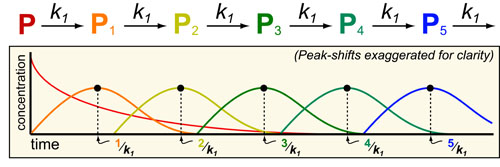In our sixth post on Understanding Kinetics, we consider pulse-chase experiments which are a common method to study bio-synthetic pathways. In pulse-chase experiments:

1. A “pulse” of labeled metabolite (P) is added to culture media.
2. The down-stream intermediates ((P1, P2, P3, etc.)) are measured (“chase“) with mass-spectrometry or radiation.

## Kinetics #5: Molecular Complexes (e.g. drug-target)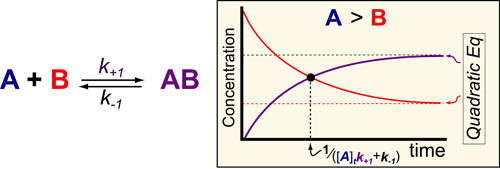In our fifth post on Understanding Kinetics, we consider the speed at which molecular complexes form. This is the fundamental mechanism underlying drug action (i.e. drugs inhibition their targets) and cellular signalling (i.e. ligands activate their receptors) and is probably the most important “kinetic effect” to consider in experimental design. Here again we use previously derived mathematical models1 to define some simple rules for the timescales/half-lives and magnitude of these reactions (figure above).

## Kinetics #4: Reversible On-Off States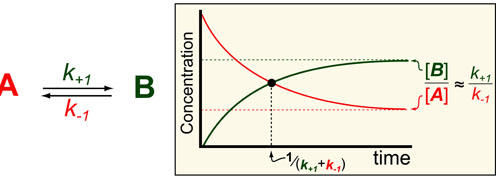In our fourth post in the Understanding Kinetics series we consider the speed at which proteins can turn off (A) or on (B). The dynamics of such processes are important to consider when designing experiments (i.e. How long should I wait to take a measurement?) and understanding Network Motifs in signalling cascades. Luckily we can use exact mathematical models (equations below1) for such processes to define some intuitive rules for the timescales/half-lives and magnitude of these reactions (figure above).

## A “Chemical-Structure Map” of the Metabolome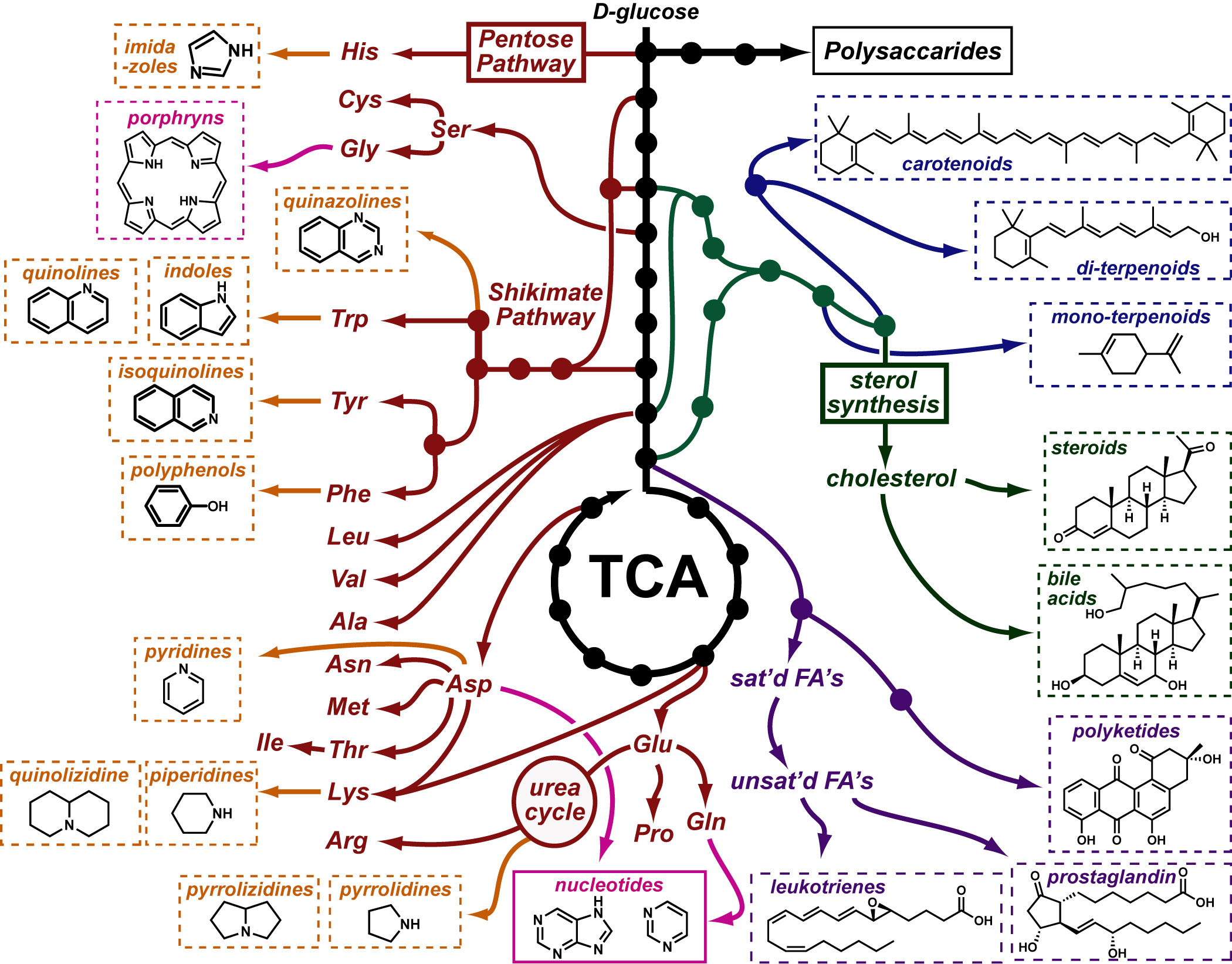I’ve always struggled to connect the structures of natural products with the biosynthetic pathways that generate them. I recently found a great resource in the Kyoto Encyclopedia of Genes and Genomes (KEGG) which helped me address this problem directly. The figure above is an adaptation of several of their pathway charts most especially that pictured here.

## An Idea for Visualizing Receptor/Ligand X-Ray Structures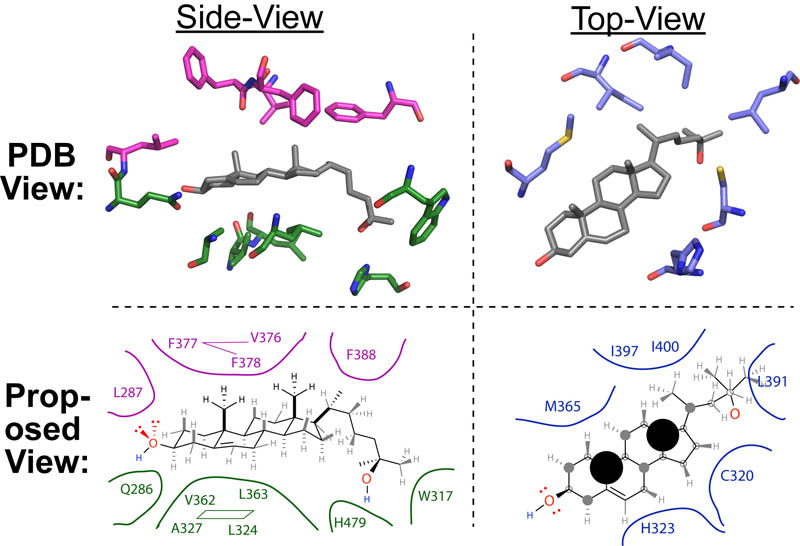As an organic chemist, I have always had difficulty relating the 3D PDB/X-ray structures of receptor/ligand complexes to the 2D chemical drawing chemist build their “chemical insight” off of. In the figure above, I present an idea to bridge the gap by presenting sets of receptor residues as “surfaces” interacting with the two types of 2D representations chemists use to think about chemistry.

## Estimating Metabolite Concentrations at Steady StateIn a follow up to our post on sequential biochemical pathways, we next wanted to present an method to approximate the concentration of a metabolic intermediate in a biosynthetic pathway. In general, under steady state conditions, the steady state concentration of a metabolite can be estimated from the ratio of the Vmax for the upstream rate-determining enzyme over the rate of decay of that metabolite. A more complete equation is detailed below and further discussed in our post on Estimating Protein/Metabolite Levels from RNAseq data.

## Kinetics #3: Branch Points in Biochemical Pathways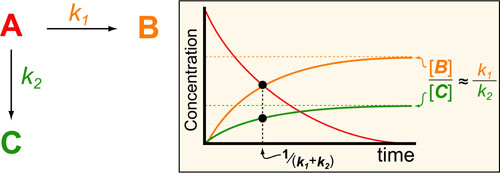In a follow up to our posts on Intuiting Enzyme Kinetics and Sequential Biochemical Pathways, we next wanted to consider the kinetic curves branch points in biochemical pathways (or equivalently kinetic competitions). Luckily exact mathematical models exist for competitive first-order processes (see below) and we can use these to develop intuitive rules (figure above) if we consider these pathways to be approximately pseudo-first order (which is often true in the context of biosynthetic pathways).

## Kinetics #2: Sequential Biochemical Pathways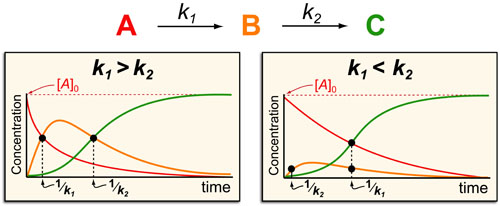In a follow up to our post our post on intuiting enzyme kinetics, we next wanted to consider the kinetic curves for sequential biochemical pathways. Luckily exact mathematical models exist for sequential first-order processes (see below) and we can use these to develop intuitive rules (figure above) if we consider these pathways to be approximately pseudo-first order (which is often true in the context of biosynthetic pathways).

## Kinetics #1: Catalyzed Reaction Timescales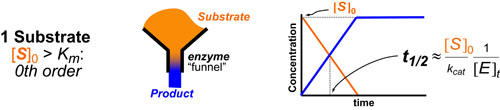Most chemical and biochemical research concerns reaction that are catalyzed by either an enzyme or a chemical catalyst. Unfortunately, when we learn chemical kinetics, it is usually in the context of idealized: uncatalyzed 0th-order, 1st-order of 2nd-order chemical reactions. Luckily the intuition we learn from 0 and 1st order processes can be readily extended to catalyzed processes by invoking the pseuso-1st order approximation. Below we describe how to “think about” the most common enzymatic/catalytic kinetic curves you may encounter.

## Binding #3: Competitive Inhibition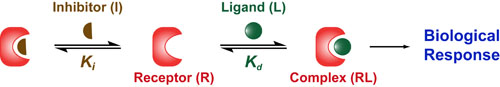While many drugs act like agonists and “turn on” their target/receptor (see posts on Hill Equation and Agonist Limits), many more drugs act like antagonists and “turn off” their target/receptor. One of the most common mechanisms by which drugs “turn off” a target receptor (R) is by blocking the binding of its natural ligand (L). This mechanism is called competitive inhibition and the antagonist is known as an inhibitor (I). Here there are two dissociation constants:the Kd which describes the strength of the interaction between R and L and the Ki which describes the strength of the interaction between R and I.

## Binding #2: The Michaelis-Menton Equation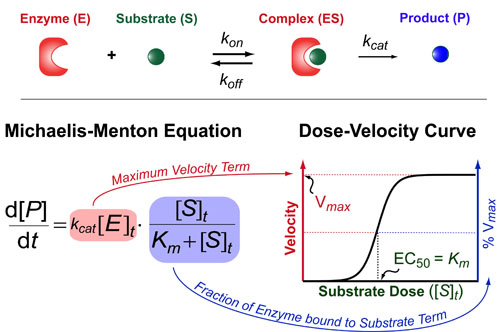The Michaelis-Menton Equation has a very similar form to the Hill Equation but the key difference is that it deals with enzyme rates not ligand/receptor or drug/target interactions per se. Basically, it describes how fast an enzyme (E) makes its product (P) as a function of the total concentration of substrate ([S]t). This rate of production formation (d[P]/dt) is proportional to the kcat and the amount of complex ([ES]) which is exact what the Michaelis-Menton equation models. The Michaelis-constant (Km = (koff+kcat) / kon) describes how tightly the substrate binds the enzyme and the kcat is a rate-constant that describes how quickly the enzyme can make the product. Here,brackets denote concentrations and a t subscript indicates “total concentrations.”

## Binding#1: Understanding the Hill Equation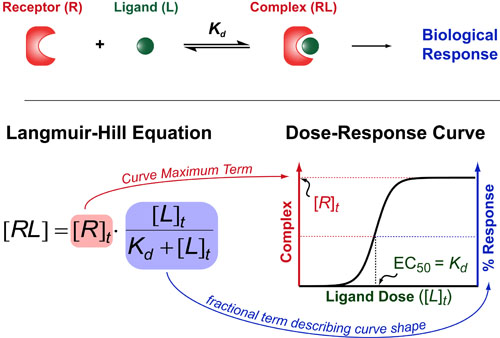If you want to understand how drugs work/behave (or just how 2 molecules interact with each other) then you need to understand one of the oldest and most useful theories in biochemistry: the Hill Equation. The Hill Equation mathematically models how Ligands(L)/Drugs interaction with their Receptors(R)/targets and generally assumes that the amount of complex that forms (RL) is proportional to some biological response . Here the Kd represents the “dissociation constant” (which is a measure of the strength of the interaction between R and L) and brackets denote concentrations/dosages (with a t subscript indicating total concentrations).

## Timescales, Kinetics, Rates, Half-lives in Biology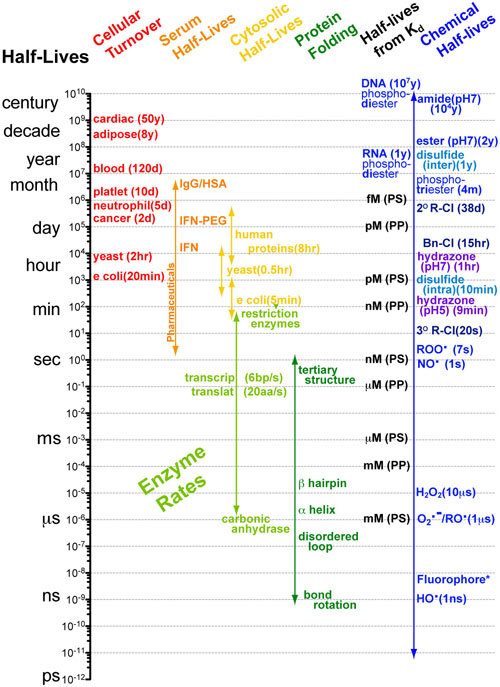Understanding interactions between processes with different rates can made significantly easier when you know the approximate timescales or half-lives for those processes.   Comparing two processes (e.g. alpha helix folding of a peptide chain (micro seconds (10-6s)) and translation of a peptide chain ( milliseconds(10-3s))) if the timescales differ by more than a factor of 100 then you can reasonably assume that as the faster process is occurring, the slower process is standing still (i.e. not occurring). Equivalently you can assume that as the slower process is occuring the faster process occurs instantly. What this means for the example cited above is that the folding of a peptide chain can and does occur before translation of that peptide chain has finished.1-3

## Understanding Ligand-Receptor Dose Response Curves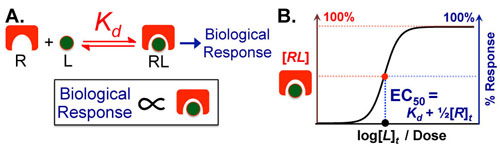Figure 1 A.
Most Ligand (L)–Receptor (R) dose–response curves are characterized by formation of a complex followed by biological effect. The underlying assumption is that biological effect is proportional to the amount of complex that forms. B. Assuming the biological effect and amount of complex formed are proportional, dose–response curves can be envisioned on two equivalent axes: amount complex (in red) and percentage biological response (in blue). The key to intuitively understanding these curves is to understand the Effective Concentration 50% or EC50 which tells you the dose of ligand necessary to elicit a 50% response. The EC50 is also known as the ligand’s potency.

Biological phenomena are often caused by the binding of a ligand (L (e.g. drug, hormone, etc.)) to a target receptor (R), which induces a biological response (Figure 1A). Typically, the biological response is proportional to the amount of ligand-receptor complex (RL) that forms (Figure 1A); as a result, the dose–response curve can have two equivalent y-axes: amount of complex (in red) or biological response (in blue). These curves have a sigmoidal (S-like) shape, where low doses of ligand have no effect and high doses plateau at maximal (100%) complex formation/biological response (Figure 1B).